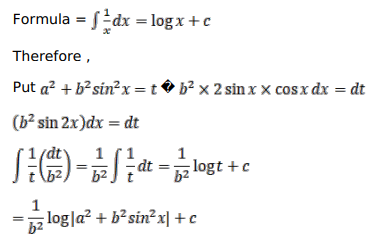# Evaluate the following integrals:Question:

Evaluate the following integrals:

$\int \frac{\sin 2 x}{\left(a^{2}+b^{2} \sin ^{2} x\right)} d x$

Solution: